# 11.6: Addition of Boron Hydrides to Alkenes. Organoboranes

$$\newcommand{\vecs}{\overset { \rightharpoonup} {\mathbf{#1}} }$$ $$\newcommand{\vecd}{\overset{-\!-\!\rightharpoonup}{\vphantom{a}\smash {#1}}}$$$$\newcommand{\id}{\mathrm{id}}$$ $$\newcommand{\Span}{\mathrm{span}}$$ $$\newcommand{\kernel}{\mathrm{null}\,}$$ $$\newcommand{\range}{\mathrm{range}\,}$$ $$\newcommand{\RealPart}{\mathrm{Re}}$$ $$\newcommand{\ImaginaryPart}{\mathrm{Im}}$$ $$\newcommand{\Argument}{\mathrm{Arg}}$$ $$\newcommand{\norm}{\| #1 \|}$$ $$\newcommand{\inner}{\langle #1, #2 \rangle}$$ $$\newcommand{\Span}{\mathrm{span}}$$ $$\newcommand{\id}{\mathrm{id}}$$ $$\newcommand{\Span}{\mathrm{span}}$$ $$\newcommand{\kernel}{\mathrm{null}\,}$$ $$\newcommand{\range}{\mathrm{range}\,}$$ $$\newcommand{\RealPart}{\mathrm{Re}}$$ $$\newcommand{\ImaginaryPart}{\mathrm{Im}}$$ $$\newcommand{\Argument}{\mathrm{Arg}}$$ $$\newcommand{\norm}{\| #1 \|}$$ $$\newcommand{\inner}{\langle #1, #2 \rangle}$$ $$\newcommand{\Span}{\mathrm{span}}$$$$\newcommand{\AA}{\unicode[.8,0]{x212B}}$$

An especially valuable group of intermediates can be prepared by addition of ancompound to carbon-carbon double or triple bonds:The reaction is called hydroboration and is a versatile synthesis of organoboron compounds. One example is the addition of diborane, $$\ce{B_2H_6}$$, to ethene. Diborane behaves as though it is in equilibrium with $$\ce{BH_3}$$ $$\left( \ce{B_2H_6} \rightleftharpoons 2 \ce{BH_3} \right)$$, and addition proceeds in three stages:The monoalkylborane, $$\ce{RBH_2}$$, and the dialkylborane, $$\ce{R_2BH}$$, seldom are isolated because they rapidly add to the alkene. These additions amount to reduction of both carbons of the double bond:Organoboranes can be considered to be organometallic compounds. Elemental boron does not have the properties of a metal, and boron-carbon bonds are more covalent than ionic. However, boron is more electropositive than either carbon or hydrogen and when bonded to carbon behaves like most metals in the sense that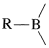bonds are polarized with $$\ce{R}$$ negative and boron positive:Hydroboration and the many uses of organoboranes in synthesis were developed largely by H. C. Brown and co-workers. In our discussion, we shall give more detail on hydroboration itself, and then describe several useful transformations of organoboranes.

## Hydroboration

The simplest borane, $$\ce{BH_3}$$, exists as the dimer, $$\ce{B_2H_6}$$, or in complexed form with certain ethers or sulfides:Any of these $$\ce{BH_3}$$ compounds adds readily to most alkenes at room temperature or lower temperatures. The reactions usually are carried out in ether solvents, although hydrocarbon solvents can be used with the borane-dimethyl sulfide complex. When diborane is the reagent, it can be generated either in situ or externally through the reaction of boron trifluoride with sodium borohydride:

$3 \overset{\oplus}{\ce{Na}} \overset{\ominus}{\ce{B}} \ce{H_4} + 4 \ce{BF_3} \rightarrow 2 \ce{B_2H_6} + 3 \overset{\oplus}{\ce{Na}} \overset{\ominus}{\ce{B}} \ce{F_4}$

Hydroborations have to be carried out with some care, because diborane and many alkylboranes are highly reactive and toxic substances; many are spontaneously flammable in air.

With unsymmetrical alkenes, hydroboration occurs so that boron becomes attached to the less-substituted end of the double bond: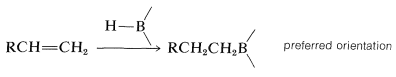Furthermore, when there is a choice, addition occurs preferentially from the less crowded side of the double bond: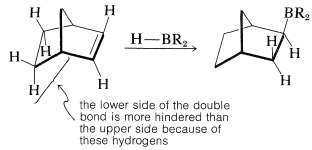If the alkene is a bulky molecule, borane may add only one or two alkene molecules to give either mono- or dialkylborane, $$\ce{RBH_2}$$ or $$\ce{R_2BH}$$, respectively, as the following reactions show:These bulky boranes still possess $$\ce{B-H}$$ bonds and can add further to a multiple bond, but they are highly selective reagents and add only if the alkene or alkyne is unhindered. This selectivity can be useful, particularly to 1-alkynes, which are difficult to stop at the alkenylborane stage when using diborane:With a bulky dialkylborane, such as di-(1,2-dimethylpropyl)borane, further addition to the alkenylborane does not occur.

An especially selective hydroborating reagent is prepared from 1,5-cyclooctadiene and borane. The product is a bicyclic compound of structure $$1$$ (often abbreviated as 9-BBN), in which the residual $$\ce{B-H}$$ bond adds to unhindered alkenes with much greater selectivity than is observed with other hydroborating reagents. It is also one of the few boranes that reacts sufficiently slowly with oxygen that it can be manipulated in air.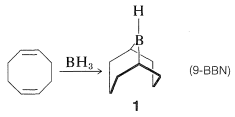An example of the difference in selectivity in the hydroboration of cis-4-methyl-2-pentene with $$\ce{B_2H_6}$$ and $$1$$ follows: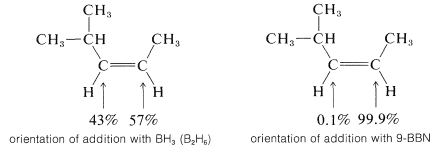## Mechanism of Hydroboration

According to the electronegativity chart (Figure 10-11), the boron-hydrogen bond is polarized in the sense $$\overset{\delta \oplus}{\ce{B}} --- \overset{\delta \ominus}{\ce{H}}$$. Therefore the direction of addition of $$\ce{B_2H_6}$$ to propene is that expected of a polar mechanism whereby the electrophilic boron atom becomes bonded to the less-substituted carbon of the double bond.

Stepwise mechanismHowever, there is no firm evidence to suggest that a carbocation intermediate is formed through a stepwise electrophilic addition reaction. For this reason, the reaction often is considered to be a four-center concerted addition.

Concerted mechanism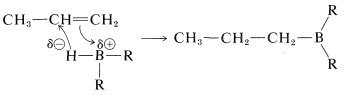The stepwise formulation explains why boron becomes attached to the less-substituted carbon, but does not account for the fact that the reactions show no other characteristics of carbocation reactions. This could be because of an expected, extraordinarily fast rate of hydride-ion transfer to the carbocation. A more serious objection to the stepwise mechanism is that alkynes react more rapidly than alkenes, something which normally is not observed for stepwise electrophilic additions (cf. Section 10-5).

## Isomerization of Alkylboranes

Some alkylboranes rearrange at elevated temperatures $$\left( 160^\text{o} \right)$$ to form more stable isomers. For example, the alkylborane $$2$$, produced by hydroboration of 3-ethyl-2-pentene, rearranges to $$3$$ on heating:In general, the boron in alkylboranes prefers to be at the end of a hydrocarbon chain so it is bonded to a primary carbon where steric crowding around boron is least severe. Thus rearrangement tends to proceed in the direction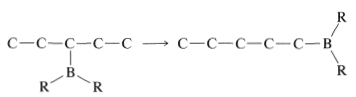Rearrangement is associated with the fact that hydroboration is reversible at elevated temperatures. This makes possible a sequence of elimination-addition reactions in which boron becomes attached to different carbons and ultimately leads to the most stable product that has boron bonded to the carbon at the end of the chain: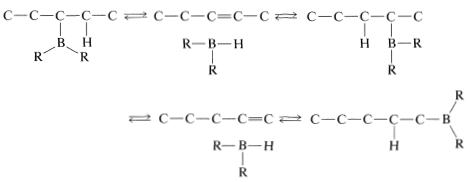Rearrangement of alkylboranes can be used to transform alkenes with double bonds in the middle of the chain into less stable 1-alkenes; for example, $$\ce{RCH=CHCH_3} \rightarrow \ce{RCH_2-CH=CH_2}$$. The procedure involves hydroboration of the starting alkene in the usual manner; the borane then is isomerized by heating. An excess of 1-decene (bp $$170^\text{o}$$) then is added to the rearranged borane and the mixture is reheated. Heating causes the alkylborane to dissociate into 1-alkene and $$\ce{HBR_2}$$; the 1-decene "scavenges" the $$\ce{HBR_2}$$ as it forms, thereby allowing a more volatile 1-alkene (bp $$<170^\text{o}$$) to be removed by simple distillation. Thus, for the rearrangement of 3-ethyl-2-pentene to 3-ethyl-1-pentene,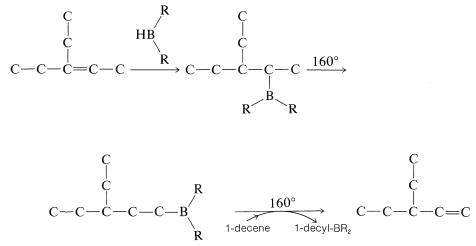## Synthetic Reactions of Organoboranes

Alkylboranes formed in the hydroboration of alkenes and alkynes seldom are isolated; for the most part they are used as reactive intermediates for the synthesis of other substances. In the reactions of alkylboranes, the $$\ce{B-C}$$ bond is cleaved in the sense $$\ce{B}^\oplus - \ce{C}^\ominus$$ so that carbon is transferred to other atoms, such as $$\ce{H}$$, $$\ce{O}$$, $$\ce{N}$$, and $$\ce{C}$$, with its bonding electron pair:In the first of these reactions (Equation 11-2), a hydrocarbon is produced by the cleavage of a borane, $$\ce{R_3B}$$, with aqueous acid, or better, with anhydrous propanoic acid, $$\ce{CH_3CH_2CO_2H}$$. The overall sequence of hydroboration-acid hydrolysis achieves the reduction of a carbon-carbon multiple bond without using hydrogen and a metal catalyst or diimide (Table 11-3):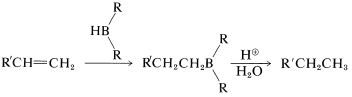Table 11-3: Some Methods of Hydrogenation of Carbon-Carbon Multiple BondsThe second reaction (Equation 11-3) achieves the synthesis of a primary alcohol by the oxidation of the alkylborane with hydrogen peroxide in basic solution. Starting with a 1-alkene, one can prepare a primary alcohol in two steps:This sequence complements the direct hydration of 1-alkenes, which gives secondary alcohols: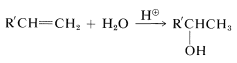Hydroboration of an alkene and subsequent reactions of the product trialkylborane, either with hydrogen peroxide or with acid, appear to be highly stereospecific. For example, 1-methylcyclopentene gives exclusively trans-2-methylcyclopentanol on hydroboration followed by reaction with alkaline hydrogen peroxide. This indicates that, overall, the reactions result in suprafacial addition of water to the double bond: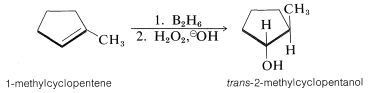Hydroboration of an alkyne followed by treatment of the alkenylborane with basic peroxide provides a method of synthesis of aldehydes and ketones. Thus hydroboration of 1-hexyne and oxidation of the 1-hexenylborane, $$4$$, with hydrogen peroxide gives hexanal by way of the enol:If $$4$$ is treated with deuteriopropanoic acid, replacement of $$\ce{-BR_2}$$ by deuterium occurs with retention of configuration, forming trans-hexene-1-$$\ce{D_1}$$: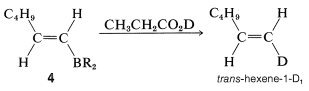## Mechanism of Oxidation of Alkylboranes

The stereospecific oxidation of alkylboranes occurs with hydrogen peroxide by an interesting and important general type of rearrangement which, for these reactions, involves migration of an organic group from boron to oxygen. The first step in the oxidation depends on the fact that tricoordinate boron has only six electrons in its valence shell and therefore behaves as if it were electron-deficient. The first step is bond formation at boron by the strongly nucleophilic peroxide anion (from $$\ce{H_2O_2} + \ce{OH}^\ominus \rightleftharpoons ^\ominus \ce{OOH} + \ce{H_2O}$$) to give a tetracovalent boron intermediate:In the second step, an alkyl group moves with its bonding electron pair from boron to the neighboring oxygen and, in so doing, displaces hydroxide ion. The stereochemical configuration of the migrating $$\ce{R}$$ group is retained: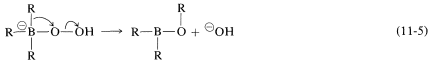Reaction is completed by hydrolysis of the $$\ce{B-O}$$ bond:All three groups on boron are replaced in this manner.

The rearrangement step (Equation 11-5) is an example of many related rearrangements in which a group, $$\ce{R}$$, migrates with its bonding electrons from one atom to an adjacent atom. We already have encountered an example in the rearrangement of carbocations (Section 8-9B):The difference between the carbocation rearrangement and the rearrangement of Equation 11-5 is that $$\ce{R}$$ migrates from boron to oxygen as $$\ce{HO}^\ominus$$ departs in what might be considered an internal $$S_\text{N}2$$ reaction. We can generalize this kind of reaction of boron with a substance, $$\ce{X-Y}$$, as in Equation 11-6:An example of the use of an $$\ce{X-Y}$$ reagent is conversion of alkylboranes to primary amines with hydroxylaminesulfonic acid, $$\ce{H_2NOSO_3H}$$ (Equation 11-4). The key steps are attack of the nucleophilic nitrogen at boron,followed by rearrangement,and hydrolysis,